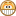## Single line JavaScript integer variable swap

May 12, 2009

In upcoming versions of ECMAScript/JavaScript, variable swapping will get a great deal easier. Many structures will start looking a lot more like Python, which is a good thing.

In Python, you can swap any variable values using the tuple notation:

`(a, b) = (b, a)`

This method is similar, but a bit more verbose, in PHP:

`list(a, b) = array(b, a);`

In yet-to-be-approved versions of ECMAScript, you'll be able to use the array syntax to swap variables:

`[a, b] = [b, a];`

So in behaviour it's closer to the PHP method, even though it looks a great deal more like the Python syntax.

Anyhoo, until these language constructs get implemented, there really is no simple way to swap variables of unknown type in JavaScript without using a third variable for storage. Using some clever math, we can swap the values for variables which we know to be integers by doing the following:

```var a = 5, b = 9;

b -= a; // a = 5, b = 4
a += b;  // a = 9, b = 4
b = a - b;  // a = 9, b = 5```

Explained in plain English: we first assign one of the variables to be the difference between the original two variables then we swing the two variables around that central point.

The downside of this method is that it's hard to conceptualize and also takes three lines of code. If we're already resigned to this swapping method being incomprehensible, is there not a way we can just compact it into a single line? The answer is yes! Just roll the above three lines into one.

```var a = 5, b = 9;

b = (a += b -= a) - b;

The important thing to note is the `- b` hanging off the end. To use this method it must come last so that the assignments earlier in the expression can change its value before it is reached.

Take for example, the following code which performs the same swapping action, but in a different order:

```var a = 5, b = 9;

a += b; // a = 14, b = 9
b = a - b; // a = 14, b = 5
a -= b; // a = 9, b = 5```

Roll these together and you get:

`a -= b = (a += b) - b;`

You'll find that this doesn't work because of the initial `-=`. If we expand that, the expression is equivalent to:

`a = a - b = (a += b) - b;`

The problem is the second `a` which has its value set before the rest of the expression. What we want to be 14, actually starts out as 5, and the end result is that `a` will equal zero. It's an interesting problem, but one that can be worked around by choosing carefully the order in which you build the expression.

If we move the `a` variable in the above equation so it comes last, then the expression will work:

`a = -(b = (a += b) - b) + a;`

Does your head a splode yet or what?Now that the final `+ a` is last, it will have the correctly assigned value when the parsing engine comes across it.

And there you have it, swapping integer variables with a single line of code in JavaScript.

 ⇐ The Brave Little Toaster: Novella vs Film Keyboard Milestone & A New Widget ⇒